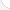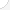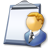Engineering technicians Description: Engineering technicians use science, engineering, and math to solve problems for businesses. They help engineers and scientists with experiments and develop models of new equipment. Some supervise production workers or check the quality of products. Like engineers, they specialize in an area such as mechanics, electronics, or chemicals. Some may be exposed to hazards from equipment, chemicals, or toxic materials.Source: Bureau of Labor Statistics, U.S. Department of Labor, Occupational Outlook Handbook, 2008-09 Edition at http://www.bls.gov/OCO/Complete Job Profile: http://www.bls.gov/oco/ocos112.htmSalary: \$50,001 or more per yearComments:

There are 49 math topics Engineering technicians need to know.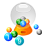Basic Math / Algebra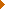FractionsDecimalsRatio and ProportionPercentCustomary MeasurementMetric MeasurementMeasurement ConversionBasic ProbabilityStatistical GraphingPowers and RootsOther Number BasesNegative NumbersScientific NotationBasic Problem Solving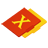First-Year AlgebraUsing FormulasLinear EquationsLinear InequalitiesOperations with PolynomialsFactoring PolynomialsRational ExpressionsCoordinate Graphing 2DQuadratic EquationsAlgebraic Representation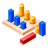GeometryBasic TerminologyAngle MeasurementTriangle InequalitiesParallel LinesQuadrilateralsSimilarityRight Triangle TrigonometryCirclesConstructionsAreaMake/Use 3D Drawings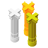Second-Year Algebra / TrigonometryVariationImaginary NumbersLogarithmsCoordinate Graphing 3DTrigonometric/Circular FunctionsOblique TrianglesPolar Coordinates/GraphsVectors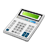Other TopicsBasic Calculator UseScientific Calculator UseComputer UseGroup Problem SolvingMental MathInductive/Deductive ReasoningMath Communications